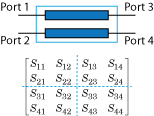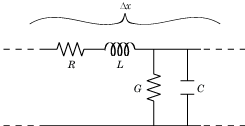# s2rlgc

Convert S-parameters to RLGC transmission line parameters

## Syntax

``rlgc_params = s2rlgc(s_params,length,freq)``
``rlgc_params = s2rlgc(___,z0)``

## Description

````rlgc_params = s2rlgc(s_params,length,freq)` transforms multi-port S-parameter data into RLGC transmission line parameters using a reference impedance of 50 Ω.```

example

````rlgc_params = s2rlgc(___,z0)` transforms multi-port S-parameter data into a frequency-domain representation of an RLGC transmission line using a characteristic impedance of `z0`. Use this option with the input arguments in the previous syntax.```

## Examples

collapse all

Define the s-parameters.

```s_11 = 0.000249791883190134 - 9.42320545953709e-005i; s_12 = 0.999250283783862 - 0.000219770154524734i; s_21 = 0.999250283783863 - 0.000219770154524756i; s_22 = 0.000249791883190079 - 9.42320545953931e-005i; s_params = [s_11,s_12; s_21,s_22];```

Specify the length, frequency of operation, and impedance of the transmission line.

```length = 1e-3; freq = 1e9; z0 = 50;```

Convert from s-parameters to rlgc-parameters.

`rlgc_params = s2rlgc(s_params,length,freq,z0)`
```rlgc_params = struct with fields: R: 50.0000 L: 1.0000e-09 G: 0.0100 C: 1.0000e-12 alpha: 0.7265 beta: 0.2594 Zc: 63.7761 -14.1268i ```

## Input Arguments

collapse all

Specify a 2N-by-2N-by-M array of S-parameters to transform into RLGC transmission line parameters. The following figure describes the port ordering convention assumed by the function.The function assumes that:

• Each 2N-by-2N matrix consists of N input terminals and N output terminals.

• The first N ports (1 through N) of the S-parameter matrix are input ports.

• The last N ports (N + 1 through 2N) are output ports.

To reorder ports before using this function, use the `snp2smp` function.

Specify the length of the transmission line in meters.

Specify the vector of M frequencies over which the S-parameter array `s_params` is defined.

Reference impedance of N-port S-Parameters, specified as positive real scalar in ohms.

## Output Arguments

collapse all

The output `rlgc_params` is structure whose fields are N-by-N-by-M arrays of transmission line parameters. Each of the M N-by-N matrices correspond to a frequency in the input vector `freq`.

• `rlgc_params.R` is an array of distributed resistances, in units of Ω/m. The matrices are real symmetric, the diagonal terms are nonnegative, and the off-diagonal terms are nonnegative.

• `rlgc_params.L` is an array of distributed inductances, in units of H/m. The matrices are real symmetric, the diagonal terms are positive, and the off-diagonal terms are nonnegative.

• `rlgc_params.G` is an array of distributed conductances, in units of S/m. The matrices are real symmetric, the diagonal terms are nonnegative, and the off-diagonal terms are nonpositive.

• `rlgc_params.C` is an array of distributed capacitances, in units of F/m. The matrices are real symmetric, the diagonal terms are positive, and the off-diagonal terms are nonpositive.

• `rlgc_params.Zc` is an array of complex characteristic line impedances, in ohms.

• `rlgc_params.alpha` is an array of real attenuation coefficients, in units of Np/m.

• `rlgc_params.beta` is an array of real phase constants, in units of rad/m.

collapse all

### RLCG Transmission Line Model

The following figure illustrates the RLGC transmission line model.The representation consists of:

• The distributed resistance, R, of the conductors, represented by a series resistor.

• The distributed inductance, L, of the conductors, represented by a series inductor.

• The distributed conductance, G, between the two conductors, represented by a shunt resistor.

• The distributed capacitance, C, between the two conductors, represented by a shunt capacitor.

RLGC component units are all per unit length Δx.

 Degerstrom, M.J., Gilbert, B.K., and Daniel, E.S . "Accurate resistance, inductance, capacitance, and conductance (RLCG) from uniform transmission line measurements."Electrical Performance of Electronic Packaging. IEEE-EPEP, 18th Conference, 27–29 October 2008, pp. 77–80.

 Sampath, M.K. "On addressing the practical issues in the extraction of RLGC parameters for lossy multi-conductor transmission lines using S-parameter models." Electrical Performance of Electronic Packaging,. IEEE-EPEP, 18th Conference, 27–29 October 2008, pp. 259–262.

 Eisenstadt, W. R., and Eo, Y. "S-parameter-based IC interconnect transmission line characterization," IEEE Transactions on Components, Hybrids, and Manufacturing Technology. Vol. 15, No. 4, August 1992, pp. 483–490.